# rmapshaper Basics

#### 2020-01-16

rmapshaper is a package which is an R wrapper around the awesome mapshaper tool by Matthew Bloch, which has both a Node.js command-line tool as well as an interactive web tool.

The main advantage of the package is the availability of the topologically-aware simplification algorithm in ms_simplify (provided by the simplify tool in mapshaper). This means that shared boundaries between adjacent polygons are always kept intact, with no gaps or overlaps, even at high levels of simplification. It uses the Visvalingam simplification method.

At this time, rmapshaper provides the following functions:

• ms_simplify - simplify polygons or lines
• ms_clip - clip an area out of a layer using a polygon layer or a bounding box. Works on polygons, lines, and points
• ms_erase - erase an area from a layer using a polygon layer or a bounding box. Works on polygons, lines, and points
• ms_dissolve - aggregate polygon features, optionally specifying a field to aggregate on. If no field is specified, will merge all polygons into one.
• ms_explode - convert multipart shapes to single part. Works with polygons, lines, and points in geojson format, but currently only with polygons and lines in the Spatial classes (not SpatialMultiPoints and SpatialMultiPointsDataFrame).
• ms_lines - convert polygons to topological boundaries (lines)
• ms_innerlines - convert polygons to shared inner boundaries (lines)
• ms_points - create points from a polygon layer
• ms_filter_fields - Remove fields from the attributes
• ms_filter_islands - Remove small detached polygons

This short vignette focuses on simplifying polygons with the ms_simplify function.

### Usage

rmapshaper works with geojson strings (character objects of class geo_json) and list geojson objects of class geo_list. These classes are defined in the geojsonio package. It also works with Spatial classes from the sp package.

We will use the states dataset from the geojsonio package and first turn it into a geo_json object:

library(geojsonio)
##
## Attaching package: 'geojsonio'
## The following object is masked from 'package:base':
##
##     pretty
library(rmapshaper)
library(sp)

states_json <- geojson_json(states, geometry = "polygon", group = "group")
## Assuming 'long' and 'lat' are longitude and latitude, respectively

For ease of illustration via plotting, we will convert to a SpatialPolygonsDataFrame:

states_sp <- geojson_sp(states_json)

## Plot the original
plot(states_sp)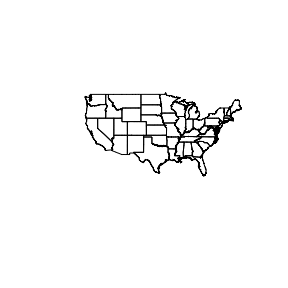Now simplify using default parameters, then plot the simplified states

states_simp <- ms_simplify(states_sp)
plot(states_simp)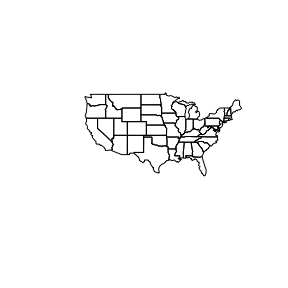You can see that even at very high levels of simplification, the mapshaper simplification algorithm preserves the topology, including shared boundaries:

states_very_simp <- ms_simplify(states_sp, keep = 0.001)
plot(states_very_simp)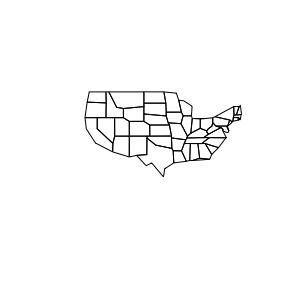Compare this to the output using rgeos::gSimplify, where overlaps and gaps are evident:

library(rgeos)
## rgeos version: 0.5-2, (SVN revision 621)
##  GEOS runtime version: 3.7.2-CAPI-1.11.2
##  Linking to sp version: 1.3-1
##  Polygon checking: TRUE
states_gsimp <- gSimplify(states_sp, tol = 1, topologyPreserve = TRUE)
plot(states_gsimp)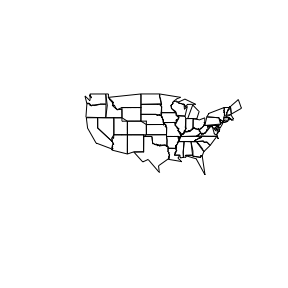The package also works with sf objects. This time we’ll demonstrate the ms_innerlines function:

library(sf)
## Linking to GEOS 3.7.2, GDAL 2.4.2, PROJ 5.2.0
states_sf <- st_as_sf(states_sp)
states_sf_innerlines <- ms_innerlines(states_sf)
plot(states_sf_innerlines)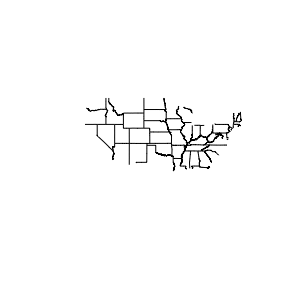All of the functions are quite fast with geo_json character objects and geo_list list objects. They are slower with the Spatial classes due to internal conversion to/from json. If you are going to do multiple operations on large Spatial objects, it’s recommended to first convert to json using geojson_list or geojson_json from the geojsonio package. All of the functions have the input object as the first argument, and return the same class of object as the input. As such, they can be chained together. For a totally contrived example, using states_sp as created above:

library(geojsonio)
library(rmapshaper)
library(sp)
library(magrittr)

## First convert 'states' dataframe from geojsonio pkg to json
states_json <- geojson_json(states, lat = "lat", lon = "long", group = "group",
geometry = "polygon")

states_json %>%
ms_erase(bbox = c(-107, 36, -101, 42)) %>% # Cut a big hole in the middle
ms_dissolve() %>% # Dissolve state borders
ms_simplify(keep_shapes = TRUE, explode = TRUE) %>% # Simplify polygon
geojson_sp() %>% # Convert to SpatialPolygonsDataFrame
plot(col = "blue") # plot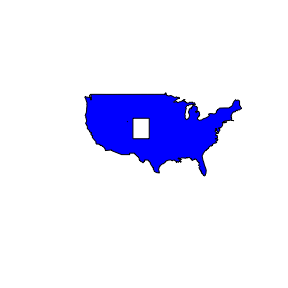### Using the system mapshaper

Sometimes if you are dealing with a very large spatial object in R, rmapshaper functions will take a very long time or not work at all. As of version 0.4.0, you can make use of the system mapshaper library if you have it installed. This will allow you to work with very large spatial objects.

First make sure you have mapshaper installed:

check_sys_mapshaper()
## mapshaper version 0.4.154 is installed and on your PATH
##  TRUE

If you get an error, you will need to install mapshaper. First install node (https://nodejs.org/en/) and then install mapshaper with:

npm install -g mapshaper

Then you can use the sys argmument in any rmapshaper function:

states_simp_internal <- ms_simplify(states_sf)
states_simp_sys <- ms_simplify(states_sf, sys = TRUE)

all.equal(states_simp_internal, states_simp_sys)
##  TRUE